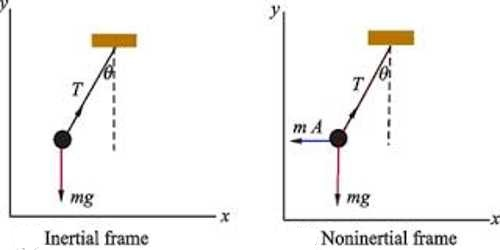# Inertial and Non-inertial Frame of Reference

Inertial and Non-inertial Frame of Reference

The frame of reference in which law of inertia and Newton’s first law of motion is applicable is called inertial frame of reference. It is also called internal frame of reference or Galilean frame. If Newton’s law of inertia is applied to the frames of reference associated with all bodies moving with all bodies moving with uniform velocity with respect to earth, each of them is also an inertial frame of reference. That means, if a frame is in uniform velocity with respect to the earth, then that frame is an inertial frame.

The decrease/increase of motion of the body, retardation/acceleration is produced, i.e., as it is not moved in the uniform velocity, so it is not an inertial frame of reference. That means, with respect to the surface of the earth if it is of uniform velocity, then the frame is an inertial frame. In the figure, the inertial frame of reference has been shown.Fig: inertial frame of reference

In this frame acceleration is,

a = d2r/dt2 = 0

Since applied force, F = ma = 0

or, d2x/dt2 = ax = 0; d2y/dt2 = ay = 0; d2z/dt2 = az = 0

Again, the frame of reference in winch law of inertia and Newton’s first law of motion is not applicable is called non-Inertial frame of reference. Rotational and reference frame moving with non-uniform velocity is non-inertial frame. In this frame acceleration occurs by hypothetical force.

Example: There is a ball inside a moving bus. If the brake is applied on the bus, it will appear that the ball is accelerated. No external force has been applied to the ball, but as we see the ball inside the bus from an accelerated frame of reference, so it appears external force is working.